# Introduction to Superpower

#### 2020-02-06

The goal of Superpower is to easily simulate single factor and factorial designs and empirically calculate power using a simulation approach. Currently, the package can only calculate power for ANOVAs. This app is intended to be utilized for prospective (a priori) power analysis. This vignette gives a brief overview of the package’s capability. If you would like to see more information on this package please see our online book

## Installation

You can install the developmental version of Superpower from GitHub with:

devtools::install_github("arcaldwell49/Superpower")

## ANOVA_design function

Currently the ANOVA_design function can create designs up three factors, for both within, between, and mixed designs. It requires the following input: design, n, mu, sd, r, and optionally allows you to set labelnames.

1. design: string that specifies the design (see below).
2. n: the sample size for each between subject condition.
3. mu: a vector with the means for each condition.
4. sd: the population standard deviation. Assumes homogeneity of variances (only one standard deviation can be provided).
5. r: the correlation for within designs (or 0 for between designs).
6. labelnames: This is an optional vector of words that indicates factor names and level names (see below).
7. A final optional setting is to specify if you want to output a plot or not (plot = TRUE or FALSE)

### Specifying the design using ‘design’

‘design’ is used to specify the design. Every factor is specified with a number, indicating the number of levels of the factor, and a letter, b or w, to indicate whether the factor is manipulated between or within participants. For example, a 2b design has two between-participant groups. A 12w design has one factor with 12 levels, all manipulated within-participants. A "2b*3w" is a design with two factors (a 2b factor and a 3w factor), the first of which has 2 between participant levels (2b), and the second of which has 3 within participants levels (3w). If there are multiple factors (the functions take up to three different factors) separate factors with a * (asterisk). An example of a 2b*3w design is a group of people in one condition who get a drug, and a group of people in another condition who get a placebo, and we measure their health before they take the pill, one day after they take the pill, and a week after they take the pill.

### Specifying the means using ‘mu’

Note that for each cell in the design, a mean must be provided. Thus, for a "2b*3w" design, 6 means need to be entered.

Means need to be entered in the correct order. ANOVA_design outputs a plot so you can check if you entered all means as you intended. Always carefully check if the plot that is generated matches your expectations.

The general principle is that the code generates factors, indicated by the factor names you entered in the labelnames variable, (i.e., condition and time). Levels are indicated by factor names and levels (e.g., control_time1, control_time2, control_time3, etc).

If your design has just one factor, just enter the means in the same order as the labelnames (see below). For more factors, note the general pattern in the example below. Means are entered in the following order for a 3 factors design (each with 2 levels):

1. a1 b1 c1
2. a1 b1 c2
3. a1 b2 c1
4. a1 b2 c2
5. a2 b1 c1
6. a2 b1 c2
7. a2 b2 c1
8. a2 b2 c2

So if you enter the means 1, 2, 3, 4, 5, 6, 7, 8 the first 4 means correspond to level 1 of factor 1, the second 4 means correspond to level 2 of factor 1. Within the first 4 means, the first 2 correspond to level 1 of factor 2, and within those 2 means, the first corresponds to level 1 of factor 3.

The plot below visualizes means from 1 to 8 being entered in a vector: mu = c(1, 2, 3, 4, 5, 6, 7, 8) so you can see how the basic ordering works.

### Specifying label names

To make sure the plots and tables with simulation results are easy to interpret, it really helps to name all factors and levels. You can enter the labels in the ‘labelnames’ variable. You can also choose not to specify names. Then all factors are indicated by letters (a, b, c) and all levels by numbers (a1, a2, a3).

For the 2x3 design we have been using as an example, where there are 2 factors (condition and time of measurement), the first with 2 levels (placebo vs. medicine) and the second with three levels (time1, time2, and time3) we would enter the labels as follows:

c(“condition”, “placebo”, “medicine”, “time”, “time1”, “time2”, “time3”)

As you can see, you follow the order of the design (2b*3w), and first write the FACTOR label (condition) followed by the levels of that factor (placebo and medicine). Then you write the second factor name (time) followed by the three labels for each LEVEL (time1, time2, time3). Do not use spaces in the names (so not “time 1” but “time1” or “time_1”).

Some examples:

1. One within factor (time with 2 levels), 2w:
• c("time", "morning", "evening")
1. Two between factors (time and group, each with 2 levels), 2b*2b:
• c("time", "morning", "evening", "group", "control", "experimental")
1. Two between factors (time and group, first with 4 levels, second with 2 levels), 4b*2b:
• c("time", "morning", "afternoon" ,"evening", "night", "group", "control", "experimental")

### Specifying the correlation

Depending on whether factors are manipulated within or between, variables are correlated, or not. You can set the correlation for within-participant factors. You can either assume all factors have the same correlation (e.g., r = 0.7), or enter the correlations for each pair of observations separately by specifying a correlation matrix.

#### Specifying the correlation matrix.

In a 2x2 within-participant design, with factors A and B, each with 2 levels, there are 6 possible comparisons that can be made.

1. A1 vs. A2
2. A1 vs. B1
3. A1 vs. B2
4. A2 vs. B1
5. A2 vs. B2
6. B1 vs. B2

The number of possible comparisons is the product of the levels of all factors squared minus the product of all factors, divided by two. For a 2x2 design where each factor has two levels, this is:

(((2*2)^2)-(2*2))/2
##  6

The number of possible comparisons increases rapidly when adding factors and levels for each factor. For example, for a 2x2x4 design it is:

(((2*2*4)^2)-(2*2*4))/2
##  120

Each of these comparisons can have their own correlation if the factor is manipulated within subjects (if the factor is manipulated between subjects the correlation is 0). These correlations determine the covariance matrix. Potvin and Schutz (2000) surveyed statistical tools for power analysis and conclude that most software packages are limited to one factor repeated measure designs and do not provide power calculations for within designs with multiple factor (which is still true for software such as G*Power). Furthermore, software solutions which were available at the time (DATASIM by Bradley, Russel, & Reeve, 1996) required researchers to assume correlations were of the same magnitude for all within factors, which is not always realistic. If you do not want to assume equal correlations for all paired comparisons, you can specify the correlation for each possible comparison.

The order in which the correlations are entered in the vector should match the covariance matrix. The order for a 2x2 design is given in the 6 item list above. The general pattern is that the matrix is filled from top to bottom, and left to right, illustrated by the increasing correlations in the table below.

Factor a1_b1 a1_b2 a2_b1 a2_b2
a1_b1 1.00 0.91 0.92 0.93
a1_b2 0.91 1.00 0.94 0.95
a2_b1 0.92 0.94 1.00 0.96
a2_b2 0.93 0.95 0.96 1.00

The diagonal is generated dynamically (and all conditions are perfectly correlated with themselves).

We would enter this correlation matrix as:

design_result <- ANOVA_design(design = "2w*2w",
n = 80,
mu = c(1.1, 1.2, 1.3, 1.4),
sd = 2,
r <- c(0.91, 0.92, 0.93, 0.94, 0.95, 0.96))

We can check the correlation matrix by asking for it from the design_result object to check if it was entered the way we wanted:

design_result$cor_mat ## a1_b1 a1_b2 a2_b1 a2_b2 ## a1_b1 1.00 0.91 0.92 0.93 ## a1_b2 0.91 1.00 0.94 0.95 ## a2_b1 0.92 0.94 1.00 0.96 ## a2_b2 0.93 0.95 0.96 1.00 Note that we did not specify the labelnames, and thus they are automatically created. ### Specifying the sample size You can set the sample size per condition by setting a value for n. The assumption is that you will collect equal sample sizes in all conditions [expanding Superpower to allow different sample sizes in each group is a planned future option]. ### Specifying the standard deviation You can set the common standard deviation by setting a value of sd. Superpower allows you to perform calculations under the assumption of homogeneity of variances (the standard deviation is the same across conditions), or you can violate this assumption by entering a vector of standard deviations (one for each condition). If multiple standard deviations are entered then we highly recommend that a null design is also simulated to see the impact of this assumption violation on type 1 error. Also, please note that there is always some uncertainty in which values you can expect in the study you are planning. It is therefore useful to perform sensitivity analyses (e.g., running the simulation with the expected standard deviation, but also with more conservative or even worst-case-scenario values). # Simulation-Based Power Calculations There are two ways to calculate the statistical power of a factorial design based on simulations. The first is to repeatedly simulate data for each condition based on the means, sample size, standard deviation, and correlation, under specific statistical assumptions (i.e., normally distributed). The ANOVA_power function allows you to perform power analyses based on repeatedly simulating normally distributed data. A second approach is to simulate a dataset that has exactly the desired properties - every cell of the design has n datapoints that have the desired mean and standard deviation, and correlation between groups (for a within design). By performing an ANOVA on this dataset, we can calculate the required statistics from the ANOVA result used to calculate the statistical power. The ANOVA_exact function allows you to calculate power based on this approach. The ANOVA_power function is a bit more flexible (e.g., we can use different ways to correct for multiple comparisons, or in future versions of the package allow you to simulate more realistic datasets that are not normally distributed), but the ANOVA_exact function is much faster (and takes seconds, instead of minutes or hours for a large number of simulations). ## The ANOVA_power function The ANOVA_power function takes the result from the ANOVA_design function, and simulates data nsims times using a specified alpha level. As output, it provides a table for the ANOVA results, and the results for all independent comparisons. It requires the following input: ANOVA_design, alpha_level, p_adjust, nsims, seed, and verbose. 1. design_result: Output from the ANOVA_design function saved as an object 2. alpha_level: Alpha level used to determine statistical significance 3. p_adjust: Correction for multiple comparisons for exploratory ANOVA/MANOVA using the p.adjust function 4. nsims: number of simulations to perform 5. seed: Set seed for reproducible results 6. verbose: Set to FALSE to not print results (default = TRUE) With the following optional input: 1. contrast_type: This input will determine the contrast type for the emmeans comparisons. The default is “pairwise”. Possible input is limited to “pairwise”, “revpairwise”, “eff”, “consec”, “poly”, “del.eff”, “trt.vs.ctrl”, “trt.vs.ctrl1”, “trt.vs.ctrlk”, and “mean_chg”. See help(“contrast-methods”) with the emmeans package loaded for more information. 2. emm_model: emmeans accepts univariate and multivariate models. This will only make a difference if a within-subjects factor is included in the design. Setting this option to “multivariate” will result in the “multivariate” model being selected for the emmeans comparisons. This is generally recommended if you are interested in the estimated marginal means (e.g., pairwise comparisons). 3. emm_comp: This selects the factors to be included for the emmeans. The default is to take the frml2 object from the results of ANOVA_design, and with the default contrast_type = “pairwise”, results in all the pairwise comparisons being performed. The simple effects can also be performed by including | in the emm_comp formula. For example, with two factors (e.g., a and b) emm_comp = "a+b" results in all pairwise comparisons being performed while emm_comp = "a|b" will result in pairwise comparisons across all levels of a within each level of b. 4. emm_p_adjust: adjustment for multiple comparisons. Note: this feature only works with ANOVA_power. Currently, this feature includes the Tukey (“tukey”), Scheffe (“scheffe”), Sidak (“sidak”), and Dunnett (“dunnettx”) adjustments in addition to all of the options for p_adjust. Simulations typically take some time. Larger numbers of simulations yield more accurate results, but also take a long time. We recommend testing the set up with 100 simulations, and run 1000 if the set-up is correct (or 10000 if you are getting a coffee, or 100.000 if you are about accuracy to digits behind the decimal). ## The ANOVA_exact function The ANOVA_exact function takes the result from the ANOVA_design function, and simulates one dataset that exactly matches the desired properties (using the mvrnorm function from the MASS package, using the setting empirical = TRUE). This dataset is used to perform a single ANOVA, and the results are used to compute power (thanks to Chris Aberson for inspiring this approach). ANOVA_exact requires the following input: ANOVA_design, alpha_level, and verbose. 1. design_result: Output from the ANOVA_design function saved as an object 2. alpha_level: Alpha level used to determine statistical significance 3. verbose: Set to FALSE to not print results (default = TRUE) Compared to the ANOVA_power function, the ANOVA_exact approach is much faster (it requires simulating only a single dataset). Currently the only difference is that ANOVA_exact does not allow you to examine the consequences of correcting for multiple comparisons (there is no p_adjust option), and that ANOVA_exact does not work for sample sizes smaller than 8 (because it is not possible to simulate a dataset with exactly the desired properties for very small sample sizes). ## An Example Imagine you plan to perform a study in which participants interact with an artificial voice assistant that sounds either like a human or like a robot, and who sounds either cheerful or sad. In the example below, 1000 simulations for a 2*2 mixed design (first factor, voice, is manipulated between participants, the second factor, emotion, is manipulated within participants) are performed. The sample size is 40 in each between subject condition (so 80 participants in total), the assumed population standard deviation is 1.03, the correlation for the within factors is 0.8, and the means are 1.03, 1.21, 0.98, 1.01. No correction for multiple comparisons is applied. The alpha level used as a significance threshold is set to 0.01 for this simulation. design_result <- ANOVA_design(design = "2b*2w", n = 40, mu = c(1.03, 1.41, 0.98, 1.01), sd = 1.03, r=0.8, labelnames = c("voice", "human", "robot", "emotion", "cheerful", "sad"), plot = TRUE)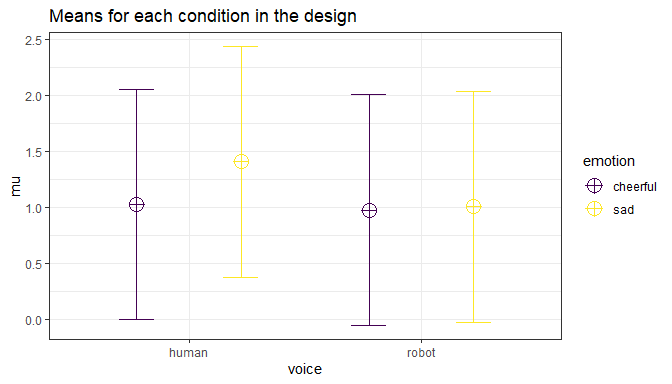power_result_vig_1 <- ANOVA_power(design_result, alpha = 0.05, nsims = nsims, seed = 1234) The result for the power simulation is printed, and has two sections (which can be suppressed by setting verbose = FALSE). The first table provides power (from 0 to 100%) and effect sizes (partial eta-squared) for the ANOVA result. We see the results for the main effects of factor voice, emotion, and the voice*emotion interaction. The result for the power simulation reveal power is highest for the main effect of emotion. Remember that this is the within-subjects factor, and the means are highly correlated (0.8) - so we have high power for within comparisons. Power is lower for the interaction, and very low for the main effect of voice. An ANOVA is typically followed up with contrasts. A statistical hypothesis often predicts not just an interaction, but also the shape of an interaction. For example, when looking at the plot of our design above, we might be specifically interested in comparing the independent effect for the cheerful vs sad human voice assistant, and the difference for sad voice when they are robotic or human. The second table provides the power for t-tests for all comparisons, and the effect sizes (Cohen’s d for between-subject contrasts, and Cohen’s $$d_z$$ for within-subject contrasts, see Lakens, 2013). Power is relatively high for the differences between within-participant conditions, and power is very low for the minor differences among the three similar means (1.03, 0.98, 1.01). In addition to the two tables, the ANOVA_power function returns the raw simulation data (all p-values and effect sizes for each simulation, see simulation_result$sim_data) and a plot showing the p-value distributions for all tests in the ANOVA.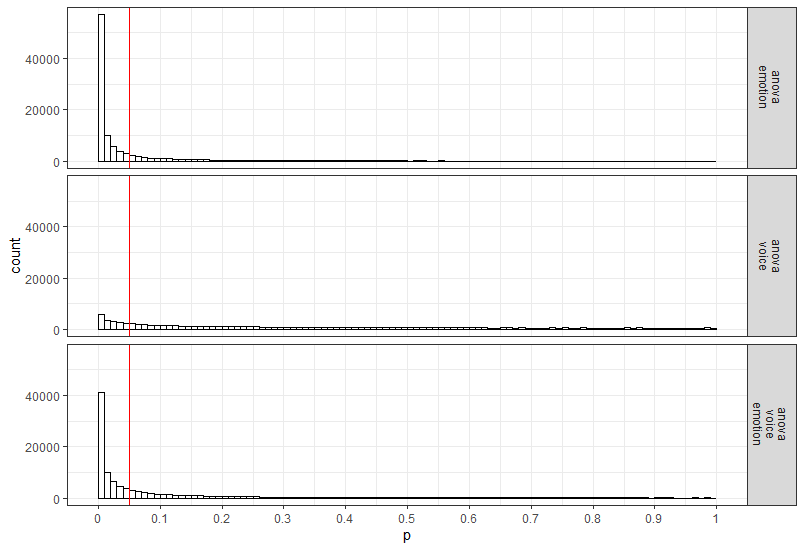## Power in a Two Group One-Way ANOVA

Imagine we aim to design a study to test the hypothesis that giving people a pet to take care of will increase their life satisfaction. We have a control condition, and a condition where people get a pet, and randomly assign participants to either condition. We can simulate a One-Way ANOVA with a specified alpha, sample size, and effect size, on see which statistical power we would have for the ANOVA. We expect pets to increase life-satisfaction compared to the control condition. Based on work by Pavot and Diener (1993) we believe that we can expect responses on the life-satisfaction scale to have a mean of approximately 24 in our population, with a standard deviation of 6.4. We expect having a pet increases life satisfaction with approximately 2.2 scale points for participants who get a pet. We plan to collect data from 200 participants in total, with 100 participants in each condition. We examine the statistical power our design would have to detect the differences we predict.

design <- "2b"
n <- 100
mu <- c(24, 26.2)
sd <- 6.4
labelnames <- c("condition", "control", "pet") #

design_result <- ANOVA_design(design = design,
n = n,
mu = mu,
sd = sd,
labelnames = labelnames)
power_result_vig_2 <- ANOVA_power(design_result, nsims = nsims, seed = 1234)
#Note we do not specify any correlation in the ANOVA_design function (default r = 0), nor do we specify an alpha in the ANOVA_power function (default is 0.05)

The result shows that we have exactly the same power for the ANOVA, as we have for the t-test. This is because when there are only two groups, these tests are mathematically identical. In a study with 100 participants per condition, we would have quite low power (around 67.7%). An ANOVA with 2 groups is identical to a t-test. For our example, Cohen’s d (the standardized mean difference) is 2.2/6.4, or d = 0.34375 for the difference between the control condition and having pets, which we can use to easily compute the expected power for these simple comparisons using the pwr package.

library(pwr)
pwr.t.test(d = 2.2/6.4,
n = 100,
sig.level = 0.05,
type="two.sample",
alternative="two.sided")$power ##  0.6768572 We can also directly compute Cohen’s f from Cohen’s d for two groups, as Cohen (1988) describes, because f = $$\frac{1}{2}d$$. So f = 0.5*0.34375 = 0.171875. And indeed, power analysis using the pwr package yields the same result using the pwr.anova.test as the power.t.test. pwr.anova.test(n = 100, k = 2, f = 0.171875, sig.level = 0.05)$power
##  0.6768572

This analysis tells us that running the study with 100 participants in each condition is quite likely to not yield a significant test result, even if our expected pattern of differences is true. This is clearly not optimal. If we perform a study, we would like to get informative results - which means we should be likely to detect effect we expect or care about, if these effects exist, and not find effects, if they are absent.

How many participants do we need for sufficient power? Given the expected difference and standard deviation, d = 0.34375, and f = 0.171875, we can increase the number of participants and run the simulation until we are content. The desired power depends on the cost of a Type 2 error. Cohen recommended to aim for 80% power, or a Type 2 error rate of 20%. I personally find a 20% Type 2 error rate too high, and not balanced enough compared to a much stricter Type 1 error rate (often by default 5%, although you should justify your alpha, for example by reducing it as a function of the sample size, or by balancing error rates). Let’s say we want to aim for 90% power.

Especially when you are increasing the sample size in your design to figure out how many participants you would need, it is much more efficient to use the ANOVA_exact function. You can see in the code below that we slowly increase the sample size and find that 180 participants give a bit more than 90% power.

design_result <- ANOVA_design(design = "2b",
n = 100,
mu = c(24, 26.2),
sd = 6.4,
labelnames = c("condition", "control", "pet"),
plot = TRUE)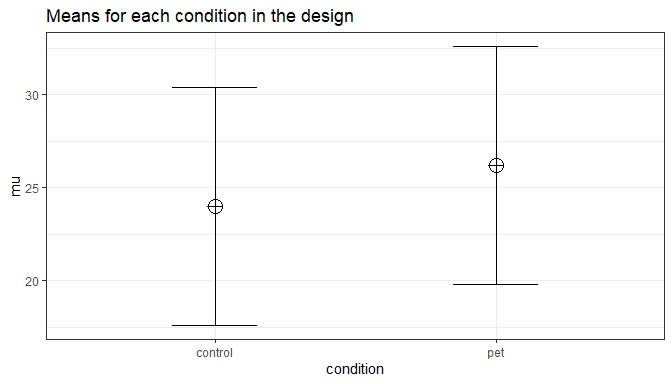ANOVA_exact(design_result,
verbose = FALSE)$main_results$power
##  67.68572
# power of 67.7 is a bit low. Let's increase it a bit to n = 150 to see if we are closer to our goal of 90% power.

design_result <- ANOVA_design(design = "2b",
n = 150,
mu = c(24, 26.2),
sd = 6.4,
labelnames = c("condition", "control", "pet"),
plot = FALSE)

ANOVA_exact(design_result,
verbose = FALSE)$main_results$power
##  84.3127
# Close, but not there yet. Let's try n = 175

design_result <- ANOVA_design(design = "2b",
n = 175,
mu = c(24, 26.2),
sd = 6.4,
labelnames = c("condition", "control", "pet"),
plot = FALSE)

ANOVA_exact(design_result,
verbose = FALSE)$main_results$power
##  89.37347
#Very close. Let's add a few more and try n = 180

design_result <- ANOVA_design(design = "2b",
n = 180,
mu = c(24, 26.2),
sd = 6.4,
labelnames = c("condition", "control", "pet"),
plot = FALSE)

ANOVA_exact(design_result,
verbose = FALSE)$main_results$power
##  90.18863

## The plot_power function

Simulation based power analyses require you to increase to sample size until power is high enough to reach your desired Type 2 error rate. To facilitate this trial and error process you can use the plot_power function to plot the power across a range of sample sizes to produce a power curve.

plot_power requires the following input: ANOVA_design, max_n, and plot.

1. design_result: Output from the ANOVA_design function saved as an object
2. min_n: The minimum sample size you want to plot in the power curve
3. max_n: The maximum sample size you want to plot in the power curve
4. plot: Set to FALSE to not print the plot (default = TRUE)

With the following optional input:

1. emm: tell the function to run emmeans or not; default is set to FALSE.
2. contrast_type: This input will determine the contrast type for the emmeans comparisons. The default is “pairwise”. Possible input is limited to “pairwise”, “revpairwise”, “eff”, “consec”, “poly”, “del.eff”, “trt.vs.ctrl”, “trt.vs.ctrl1”, “trt.vs.ctrlk”, and “mean_chg”. See help(“contrast-methods”) with the emmeans package loaded for more information.
3. emm_model: emmeans accepts univariate and multivariate models. This will only make a difference if a within-subjects factor is included in the design. Setting this option to “multivariate” will result in the “multivariate” model being selected for the emmeans comparisons. This is generally recommended if you are interested in the estimated marginal means.
4. emm_comp: This selects the factors to be included for the emmeans. The default is to take the frml2 object from the results of ANOVA_design, and with the default contrast_type = "pairwise", results in all the pairwise comparisons being performed. The simple effects can also be perfomed by including | in the emm_comp formula. For example, with two factors (e.g., a and b) emm_comp = "a+b" results in all pairwise comparisons being performed while emm_comp = "a|b" will result in pairwise comparisons across all levels of a within each level of b.

The plot_power functions simulates power up to a sample size of max_n using the ANOVA_exact function. Although it is relatively fast, with large sample size to can take some time to produce. In the figure below we can easily see that, assuming the true pattern of means and standard deviations represents our expected pattern of means and standard deviations, we have 80% power around 135 participants per condition, 90% power around 180 participants per condition, and 95% power around 225 participants per condition.

plot_power(design_result, min_n = 10, max_n = 250)

Because the true pattern of means is always unknown, it is sensible to examine the power across a range of scenarios. For example, is the difference in means is somewhat smaller,because the means are 24 and 26 instead of 24 and 26.2, we can compare the power curve above with the power curve for a slightly less optimistic scenario (where the true effect size is slightly smaller).

design_result <- ANOVA_design(design = "2b",
n = 180,
mu = c(24, 26),
sd = 6.4,
labelnames = c("condition", "control", "pet"),
plot = FALSE)

plot_power(design_result, max_n = 250)

It could be that in addition to a slightly smaller effect size, the standard deviation is slightly larger than we expected as well. This will reduce the power even further, and is thus an even less optimistic scenario. Let’s assume the true standard deviation is 6.8 instead of 6.4.

design_result <- ANOVA_design(design = "2b",
n = 180,
mu = c(24, 26),
sd = 6.8,
labelnames = c("condition", "control", "pet"),
plot = FALSE)

plot_power(design_result, min_n = 10, max_n = 250)

As these different plots make clear, your study never really has a known statistical power. Because the true effect size (i.e., the pattern of means and standard deviations) is unknown, the true power of your study is unknown. A study has 90% power assuming a specific effect size, but if the effect size is different than what you expected, the true power can be either higher or lower. We should therefore always talk about the ‘expected’ power when we do an a-priori power analysis, and provide a good justification for our expectations (i.e., for the pattern of means, standard deviations, and correlations for within designs).

## Three between subject conditions

Imagine we aim to design a study to test the hypothesis that giving people a pet to take care of will increase their life satisfaction. We have a control condition, a ‘cat’ pet condition, and a ‘dog’ pet condition. We can simulate a One-Way ANOVA with a specified alpha, sample size, and effect size, and see the statistical power we would have for the ANOVA and the follow-up comparisons. We expect all pets to increase life-satisfaction compared to the control condition. Obviously, we also expect the people who are in the ‘dog’ pet condition to have even greater life-satisfaction than people in the ‘cat’ pet condition. Based on work by Pavot and Diener (1993) we again assume that we can expect responses on the life-satisfaction scale to have a mean of approximately 24 in our population, with a standard deviation of 6.4. We expect having a pet increases life satisfaction with approximately 2.2 scale points for participants who get a cat, and 2.6 scale points for participants who get a dog. We initially consider collecting data from 150 participants in total, with 50 participants in each condition. But before we proceed with the data collection, we examine the statistical power our design would have to detect the differences we predict.

design <- "3b"
n <- 50
mu <- c(24, 26.2, 26.6)
sd <- 6.4
labelnames <- c("condition", "control", "cat", "dog") #

design_result <- ANOVA_design(design = design,
n = n,
mu = mu,
sd = sd,
labelnames = labelnames,
plot = TRUE)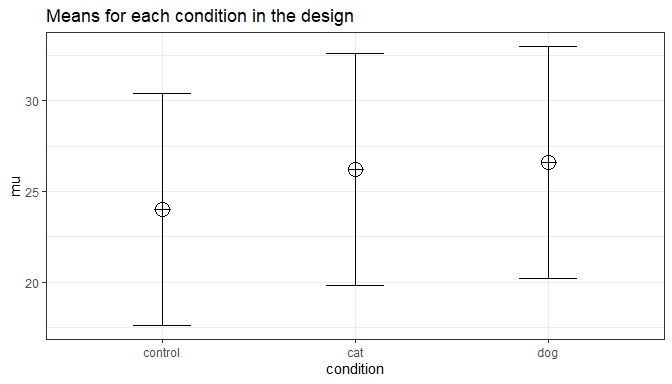ANOVA_exact(design_result,
emm = TRUE) # Pairwise emmeans will automatically run
## Power and Effect sizes for ANOVA tests
##             power partial_eta_squared cohen_f non_centrality
## condition 47.6947              0.0315  0.1804         4.7852
##
## Power and Effect sizes for pairwise comparisons (t-tests)
##                                   power effect_size
## p_condition_control_condition_cat 39.83        0.34
## p_condition_control_condition_dog 52.05        0.41
## p_condition_cat_condition_dog      6.10        0.06
##
## Power and Effect sizes for estimated marginal means
##                            contrast power partial_eta_squared cohen_f
## 1 condition_cat - condition_control 40.05              0.0197  0.1418
## 2     condition_cat - condition_dog  6.11              0.0007  0.0258
## 3 condition_control - condition_dog 52.32              0.0273  0.1675
##   non_centrality
## 1         2.9541
## 2         0.0977
## 3         4.1260

The result shows that you would have quite low power with 50 participants, both for the overall ANOVA (just below 50% power), as for the follow up comparisons (approximately 40% power for the control vs cat condition, just above 50% for the control vs dogs condition, and a really low power (around 6%, just above the Type 1 error rate of 5%) for the expected difference between cats and dogs. We can easily confirm the expected power for these simple comparisons using the pwr package. For our example, Cohen’s d (the standardized mean difference) is 2.2/6.4, or d = 0.34375 for the difference between the control condition and cats, 2.6/6.4 of d = 0.40625 for the difference between the control condition and dogs, and 0.4/6.4 or d = 0.0625 for the difference between cats and dogs as pets.

pwr.t.test(d = 2.2/6.4,
n = 50,
sig.level = 0.05,
type="two.sample",
alternative="two.sided")$power ##  0.3983064 pwr.t.test(d = 2.6/6.4, n = 50, sig.level = 0.05, type="two.sample", alternative="two.sided")$power
##  0.5205162
pwr.t.test(d = 0.4/6.4,
n = 50,
sig.level = 0.05,
type="two.sample",
alternative="two.sided")\$power
##  0.06104044

It is clear we would need to collect more participants in each group to increase our power. Let’s plot a power curve across a range of sample sizes to see how much we need to increase the sample size.

plot_power(design_result, min_n = 10, max_n = 250)

We see 134 participants give us around 90% power for the ANOVA. But we should also check the power for the comparison of the control condition and the cat condition.

design <- "3b"
n <- 134
mu <- c(24, 26.2, 26.6)
sd <- 6.4
labelnames <- c("condition", "control", "cat", "dog") #

design_result <- ANOVA_design(design = design,
n = n,
mu = mu,
sd = sd,
labelnames = labelnames,
plot = FALSE) #do not print the plot (same as above)

ANOVA_exact(design_result,
emm = TRUE)
## Power and Effect sizes for ANOVA tests
##             power partial_eta_squared cohen_f non_centrality
## condition 90.1767              0.0311  0.1793        12.8242
##
## Power and Effect sizes for pairwise comparisons (t-tests)
##                                   power effect_size
## p_condition_control_condition_cat 80.05        0.34
## p_condition_control_condition_dog 91.20        0.41
## p_condition_cat_condition_dog      8.03        0.06
##
## Power and Effect sizes for estimated marginal means
##                            contrast power partial_eta_squared cohen_f
## 1 condition_cat - condition_control 80.15              0.0195  0.1409
## 2     condition_cat - condition_dog  8.03              0.0007  0.0256
## 3 condition_control - condition_dog 91.27              0.0270  0.1665
##   non_centrality
## 1         7.9170
## 2         0.2617
## 3        11.0576
n <- 180
design_result <- ANOVA_design(design = design,
n = n,
mu = mu,
sd = sd,
labelnames = labelnames,
plot = FALSE) #do not print the plot (same as above)

ANOVA_exact(design_result,
emm = TRUE)
## Power and Effect sizes for ANOVA tests
##             power partial_eta_squared cohen_f non_centrality
## condition 96.7794              0.0311  0.1791        17.2266
##
## Power and Effect sizes for pairwise comparisons (t-tests)
##                                   power effect_size
## p_condition_control_condition_cat 90.19        0.34
## p_condition_control_condition_dog 97.02        0.41
## p_condition_cat_condition_dog      9.09        0.06
##
## Power and Effect sizes for estimated marginal means
##                            contrast power partial_eta_squared cohen_f
## 1 condition_cat - condition_control 90.24              0.0194  0.1407
## 2     condition_cat - condition_dog  9.10              0.0007  0.0256
## 3 condition_control - condition_dog 97.04              0.0269  0.1663
##   non_centrality
## 1        10.6348
## 2         0.3516
## 3        14.8535
n <- 5380
design_result <- ANOVA_design(design = design,
n = n,
mu = mu,
sd = sd,
labelnames = labelnames,
plot = FALSE) #do not print the plot (same as above)

ANOVA_exact(design_result,
emm = TRUE)
## Power and Effect sizes for ANOVA tests
##           power partial_eta_squared cohen_f non_centrality
## condition   100              0.0309  0.1786       514.8828
##
## Power and Effect sizes for pairwise comparisons (t-tests)
##                                   power effect_size
## p_condition_control_condition_cat   100        0.34
## p_condition_control_condition_dog   100        0.41
## p_condition_cat_condition_dog        90        0.06
##
## Power and Effect sizes for estimated marginal means
##                            contrast power partial_eta_squared cohen_f
## 1 condition_cat - condition_control   100              0.0193  0.1403
## 2     condition_cat - condition_dog    90              0.0007  0.0255
## 3 condition_control - condition_dog   100              0.0268  0.1659
##   non_centrality
## 1       317.8613
## 2        10.5078
## 3       443.9551

With 134 participants per condition, power for this simple comparison is only 80%. If we want to aim for 90% power for follow up tests, we need more participants. 180 participants provides good power for the follow-up comparison between control and cats. But power for the difference between cats and dogs is incredibly low. We would need 5380 participants to have 90% power for that comparison. This is possible if you can easily collect data, but might make this specific question impossible to reliably answer if data collection is costly. Realizing you can not reliably study a question is an important aspect of a-priori power analysis.

## Power in Repeated Measures ANOVA

In a repeated measures design multiple observations are collected from the same participants. In the simplest case, where there are two repeated observations, a repeated measures ANOVA equals a dependent or paired t-test. Let’s explore the impact of this correlation on the power of a repeated measures ANOVA.

### Two within conditions

To illustrate the effect of correlated observations, we start by simulating data for a medium effect size for a dependent (or paired, or within-subject) t-test. Let’s first look at G*power. If we want to perform an a-priori power analysis, we are asked to fill in the effect size $$d_z$$. As Cohen (1988) writes, “The Z subscript is used to emphasize the fact that our raw score unit is no longer X or Y, but Z”, where Z are the difference scores of X-Y.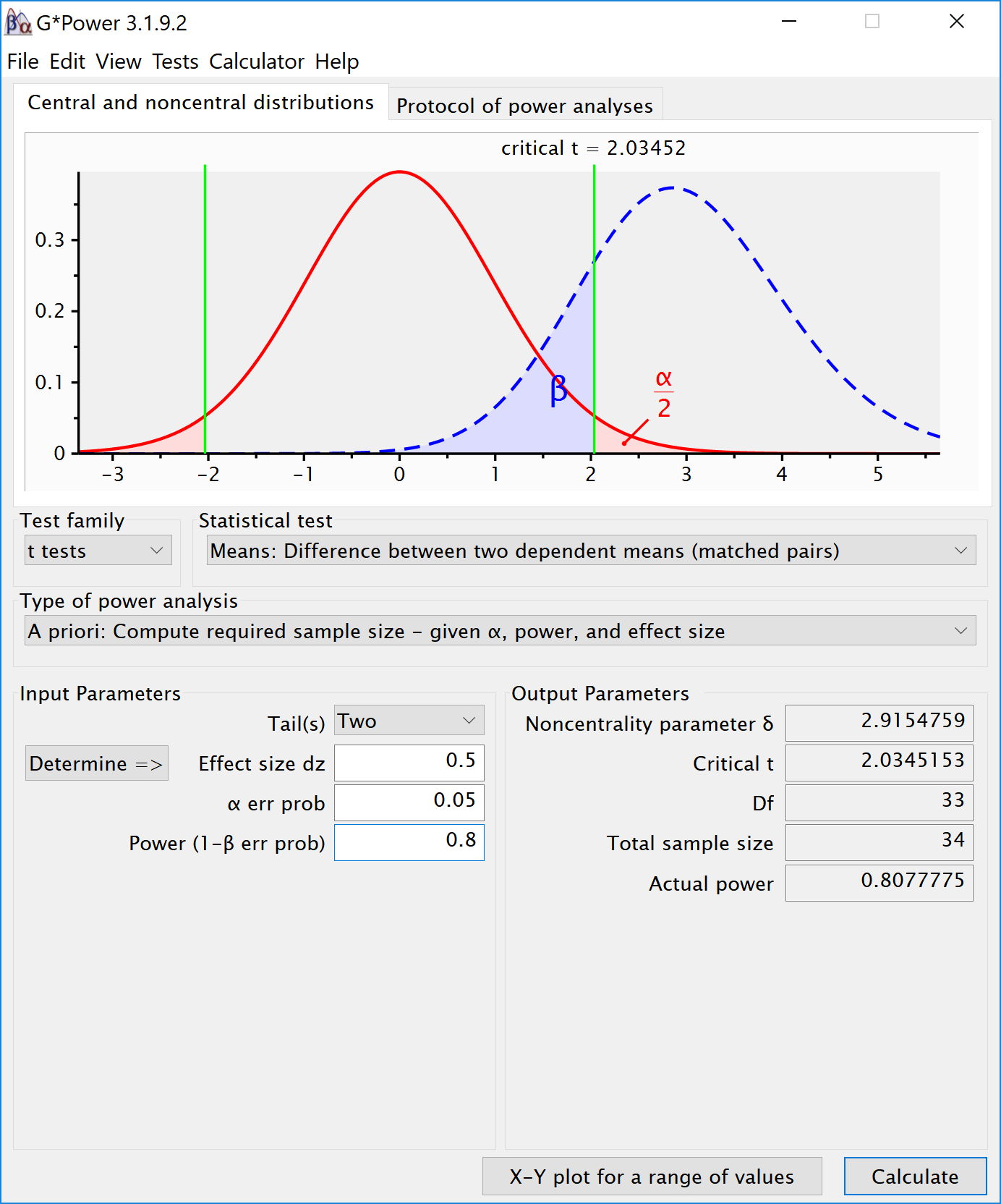Within designs can have greater power to detect differences than between designs because the values are correlated, and a within design requires less participants because each participant provides multiple observations. One difference between an independent t-test and a dependent t-test is that an independent t-test has 2(n-1) degrees of freedom, while a dependent t-test has (n-1) degrees of freedom. The sample size needed in a two-group within-design (NW) relative to the sample needed in two-group between-designs (NB), assuming normal distributions, and ignoring the difference in degrees of freedom between the two types of tests, is (from Maxwell & Delaney, 2004, p. 561, formula 45):

$$N_{W}=\frac{N_{B}(1-\rho)}{2}$$

The division by 2 in the equation is due to the fact that in a two-condition within design every participant provides two data-points. The extent to which this reduces the sample size compared to a between-subject design depends on the correlation (r) between the two dependent variables, as indicated by the 1-r part of the equation. If the correlation is 0, a within-subject design needs half as many participants as a between-subject design (e.g., 64 instead 128 participants), simply because every participants provides 2 datapoints. The higher the correlation, the larger the relative benefit of within designs, and whenever the correlation is negative (up to -1) the relative benefit disappears.

Whereas in an independent t-test the two observations are uncorrelated, in a within design the observations are correlated. This has an effect on the standard deviation of the difference scores. In turn, because the standardized effect size is the mean difference divided by the standard deviation of the difference scores, the correlation has an effect on the standardized mean difference in a within design, Cohen’s $$d_z$$. The relation, as Cohen (1988, formula 2.3.7) explains, is:

$$\sigma_{z}=\sigma\sqrt{2(1-\rho)}$$

Therefore, the relation between dz and d is $$\sqrt{2(1-\rho)}$$. As Cohen (1988) writes: "In other words, a given difference between population means for matched (dependent) samples is standardized by a value which is $$\sqrt{2(1-\rho)}$$ as large as would be the case were they independent. If we enter a correlation of 0.5 in the formula, we get $$\sqrt{2(0.5)}=1$$. When the correlation is 0.5, d = $$d_z$$. When there is a strong correlation between dependent variables, for example r = 0.9, we get $$d=d_{z}\sqrt{2(1-0.9)}$$, and a $$d_z$$ of 1 would be a d = 0.45. Reversely, $$d_{z}=\frac{d}{\sqrt{2(1-r)}}$$, so with a r = 0.9, a d of 1 would be a $$d_z$$ = 2.24. Some consider this increase in $$d_z$$ compared to d when observations are strongly correlated an ‘inflation’ when estimating effect sizes, but since the reduction in the standard deviation of the difference scores due to the correlation makes it easier to distinguish signal from noise in a hypothesis test, it leads to a clear power benefit.

There is no equivalent $$f_z$$ for Cohen’s f for a within subject ANOVA. For two groups, we can directly compute Cohen’s f from Cohen’s d, as Cohen (1988) describes, because f = $$\frac{1}{2}d$$. For a d = 0.5, f = 0.25. In G*power we can run a 2 group within-subject power analysis for ANOVA. We plan for 80% power, and reproduce the analysis above for the dependent t-test. This works because the correlation is set to 0.5, when d = $$d_z$$, and thus the transformation of f=$$\frac{1}{2}d$$ works.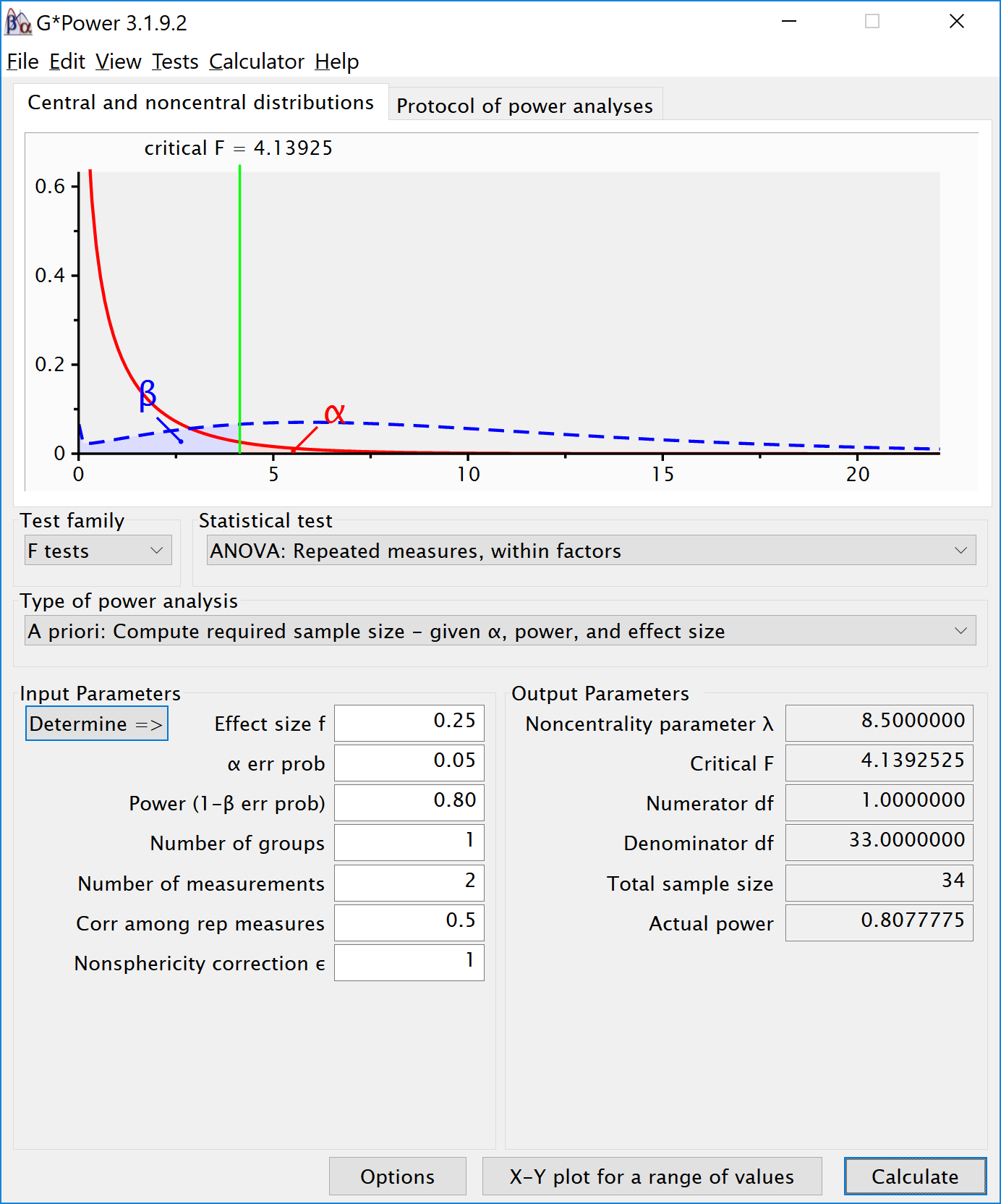If we change the correlation to 0.7 and keep all other settings the same, the repeated measure a-priori power analysis yields a sample of 21. The correlation increases the power for the test.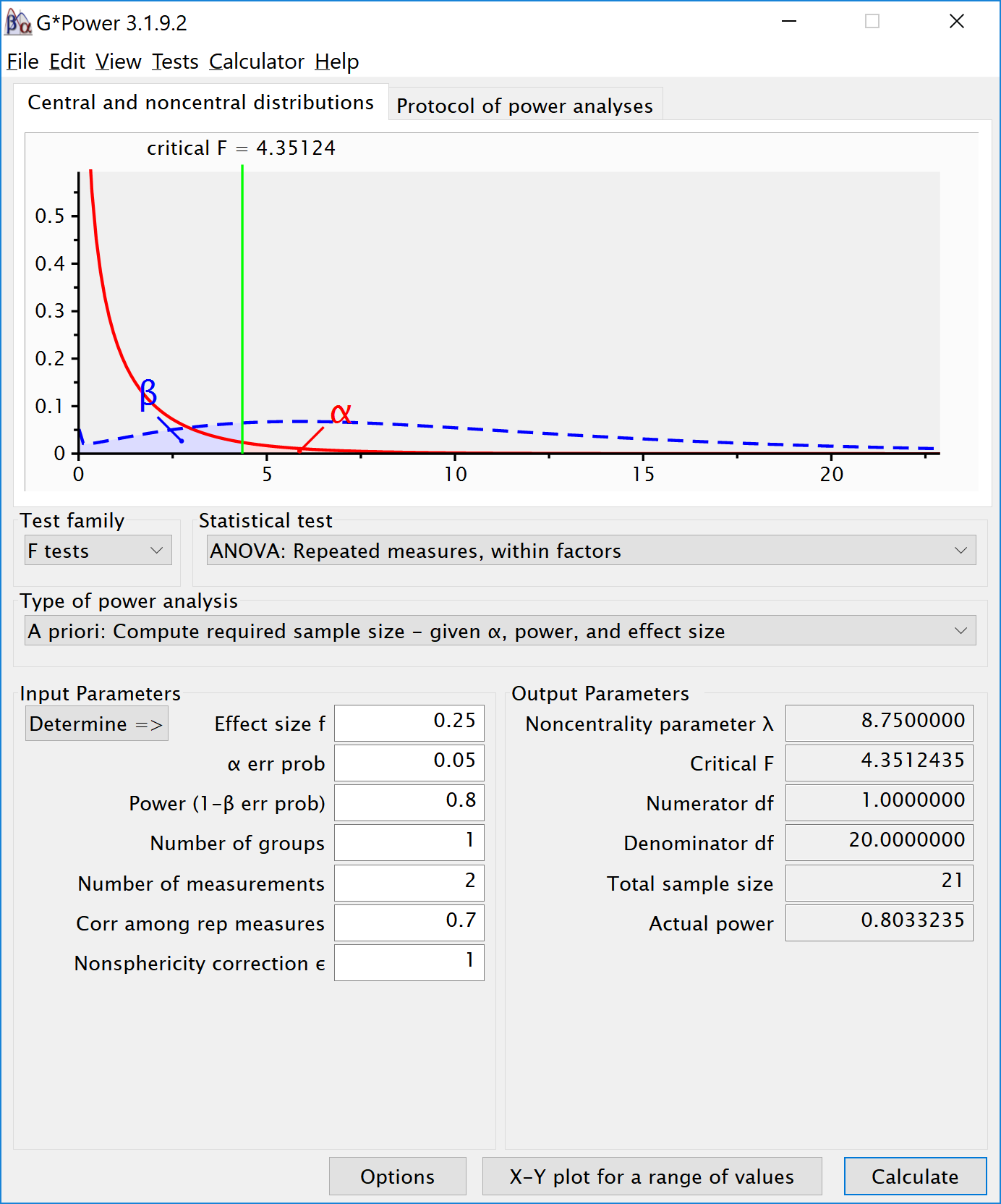To reproduce this analysis in G*power with a dependent t-test we need to change $$d_z$$ following the formula above, $$d_{z}=\frac{0.5}{\sqrt{2(1-0.7)}}$$, which yields $$d_z$$ = 0.6454972. If we enter this value in G*power for an a-priori power analysis, we get the exact same results (as we should, since an repeated measures ANOVA with 2 groups equals a dependent t-test). This example illustrates that the correlation between dependent variables always factors into a power analysis, both for a dependent t-test, and for a repeated measures ANOVA. Because a dependent t-test uses $$d_z$$ the correlation might be less visible, but given the relation between d and $$d_z$$, the correlation is always taken into account and can greatly improve power for within designs compared to between designs.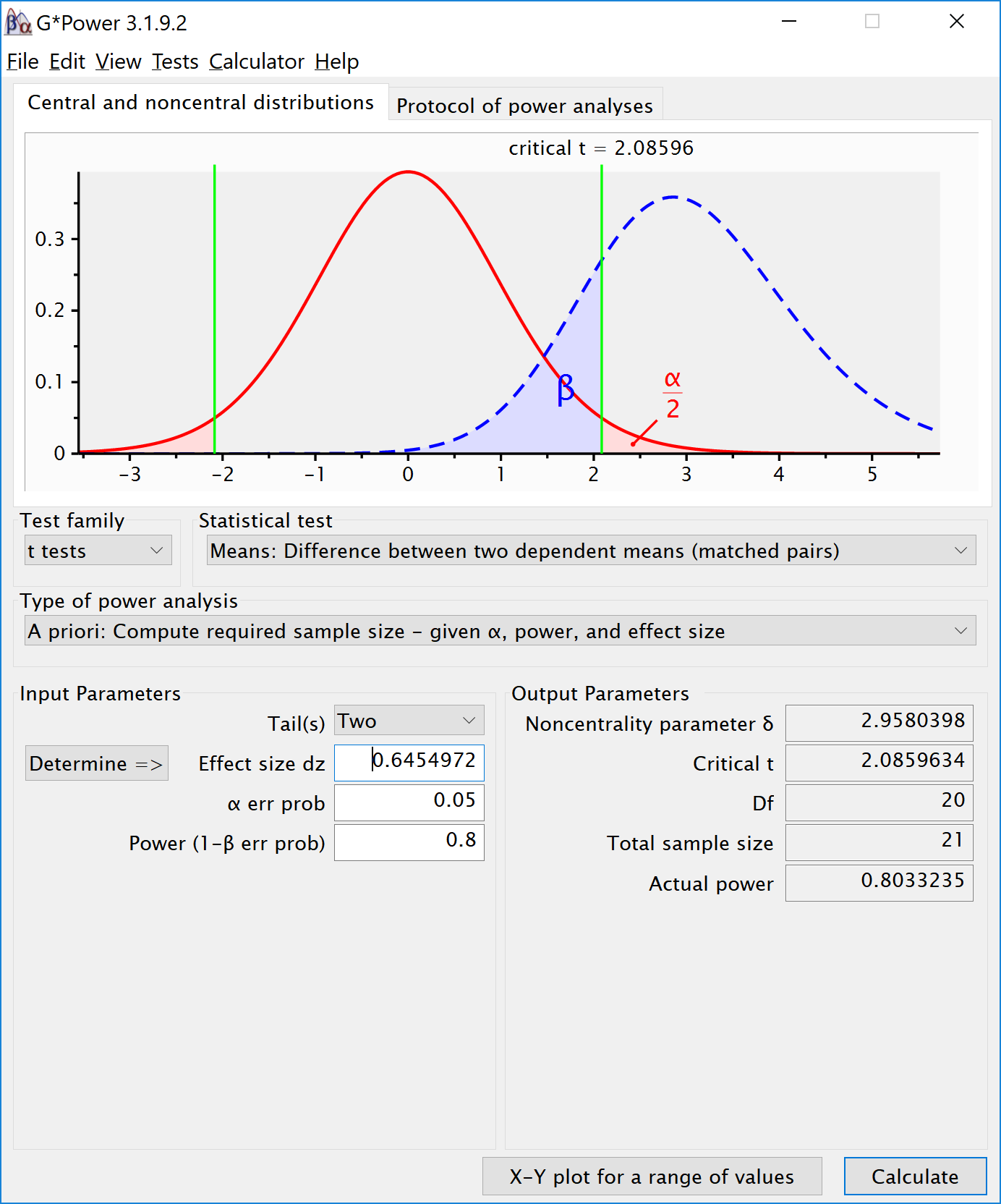We can perform both these power analyses using simulations as well. We set groups to 2 for the simulation, n = 34 (which should give 80.777 power, according to g*power), a correlation among repeated measures of 0.5, and an alpha of 0.05. In this case, we simulate data with means -0.25 and 0.25, and set the sd to 1. This means we have a mean difference of 0.5, and a Cohen’s d of 0.5/1 = 0.5. In the first example, we set the correlation to 0.5, and the result should be 80.777% power, and an effect size estimate of 0.5 for the simple effect. We also calculate partial eta-squared for the ANOVA, which equals $$\frac{f^2}{f^2+1}$$, or 0.05882353.

K <- 2
n <- 34
sd <- 1
r <- 0.5
alpha = 0.05
f <- 0.25
f2 <- f^2
ES <- f2/(f2+1)
ES
##  0.05882353
mu <- mu_from_ES(K = K, ES = ES)
design = paste(K,"w",sep="")
labelnames <- c("speed", "fast", "slow")

design_result <- ANOVA_design(design = design,
n = n,
mu = mu,
sd = sd,
r = r,
labelnames = labelnames,
plot = TRUE)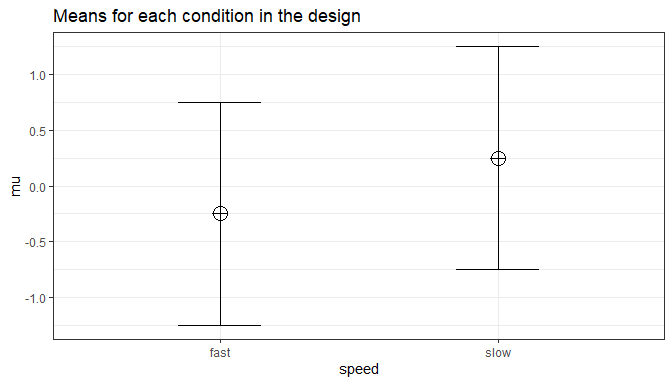ANOVA_exact(design_result,
emm = TRUE)
## Power and Effect sizes for ANOVA tests
##         power partial_eta_squared cohen_f non_centrality
## speed 80.7778              0.2048  0.5075            8.5
##
## Power and Effect sizes for pairwise comparisons (t-tests)
##                         power effect_size
## p_speed_fast_speed_slow 80.78         0.5
##
## Power and Effect sizes for estimated marginal means
##                  contrast power partial_eta_squared cohen_f non_centrality
## 1 speed_fast - speed_slow 80.78              0.2048  0.5075            8.5

The results of the exact simulation approach are indeed to 80.777%. Note that the simulation calculates Cohen’s $$d_z$$ effect sizes for paired comparisons - which here given the correlation of 0.5 is also 0.5 for a medium effect size.

We should see a larger $$d_z$$ if we increase the correlation, keeping the sample size the same, following the example in Gpower above. We repeat the simulation, and the only difference is a correlation between dependent variables of 0.7. This should yield an effect size $$d_z$$ = 0.6454972.

K <- 2
n <- 34
sd <- 1
r <- 0.7
alpha = 0.05
f <- 0.25
f2 <- f^2
ES <- f2/(f2+1)
ES
##  0.05882353
mu <- mu_from_ES(K = K, ES = ES)
design = paste(K,"w",sep="")
labelnames <- c("speed", "fast", "slow")

design_result <- ANOVA_design(design = design,
n = n,
mu = mu,
sd = sd,
r = r,
labelnames = labelnames,
plot = TRUE)ANOVA_exact(design_result,
emm = TRUE)
## Power and Effect sizes for ANOVA tests
##         power partial_eta_squared cohen_f non_centrality
## speed 95.4703              0.3004  0.6552        14.1667
##
## Power and Effect sizes for pairwise comparisons (t-tests)
##                         power effect_size
## p_speed_fast_speed_slow 95.47        0.65
##
## Power and Effect sizes for estimated marginal means
##                  contrast power partial_eta_squared cohen_f non_centrality
## 1 speed_fast - speed_slow 95.47              0.3004  0.6552        14.1667

### Power in Repeated Measures ANOVA with More than 2 Groups

We will examine a repeated measures experiment with 3 within-participant conditions, to illustrate how a repeated measures ANOVA extends a dependent t-test. In the example for a two-group within design we provided a specific formula for the sample size benefit for two groups. The sample size needed in within-designs (NW) with more than 2 conditions, relative to the sample needed in between-designs (NB), assuming normal distributions and compound symmetry, and ignoring the difference in degrees of freedom between the two types of tests, is (from Maxwell & Delaney, 2004, p. 562, formula 47):

$$N_{W}=\frac{N_{B}(1-\rho)}{a}$$

Where a is the number of within-subject levels.

### The relation between Cohen’s f and Cohen’s d

Whereas in the case of a repeated measures ANOVA with 2 groups we could explain the principles of a power analysis by comparing our test against a t-test and Cohen’s d, this becomes more difficult when we have more than 2 groups. It is more useful to explain how to directly calculate Cohen’s f, the effect size used in power analyses for ANOVA. Cohen’s f is calculated following Cohen, 1988, formula 8.2.1 and 8.2.2:

$$f = \sqrt{\frac{\frac{\sum(\mu-\overline{\mu})^2)}N}\sigma}$$

Imagine we have a within-subject experiment with 3 conditions. We ask people what they mood is when their alarm clock wakes them up, when they wake up naturally on a week day, and when they wake up naturally on a weekend day. Based on pilot data, we expect the means (on a 7 point validated mood scale) are 3.8, 4.2, and 4.3. The standard deviation is 0.9, and the correlation between the dependent measurements is 0.7. We can calculate Cohen’s f for the ANOVA, and Cohen’s dz for the contrasts:

mu <- c(3.8, 4.2, 4.3)
sd <- 0.9
f <- sqrt(sum((mu-mean(mu))^2)/length(mu))/sd #Cohen, 1988, formula 8.2.1 and 8.2.2
f
##  0.2400274
r <- 0.7
(4.2-3.8)/0.9/sqrt(2*(1-r))
##  0.5737753
(4.3-3.8)/0.9/sqrt(2*(1-r))
##  0.7172191
(4.3-4.2)/0.9/sqrt(2*(1-r))
##  0.1434438

The relation between Cohen’s d or dz and Cohen’s f becomes more difficult when there are multiple groups, because the relationship depends on the pattern of the means. Cohen (1988) presents calculations for three patterns, minimal variability (for example, for 5 means: -0.25, 0, 0, 0, 0.25), medium variability (for example, for 5 means: -0.25, -0.25, 0.25, 0.25, 0.25 or -0.25, -0.25, -0.25, 0.25, 0.25). For these three patterns, formula’s are available that compute Cohen’s f from Cohen’s d, where d is the effect size calculated for the difference between the largest and smallest mean (if the largest mean is 0.25 and the smallest mean is -0.25, 0.25 - -0.25 = 0.5, so d is 0.5 divided by the standard deviation of 0.9). In our example, d would be (4.3-3.8)/0.9 = 0.5555556. If we divide this value by sqrt(2*(1-r)) we have dz = 0.5555556/0.7745967 = 0.7172191. If the means and standard deviation is known, it is best to calculate Cohen’s f directly from these values.

We can perform power analyses for within designs using simulations. We set groups to 3 for the simulation, n = 20, and the correlation between dependent variables to 0.8. If the true effect size is f = 0.25, and the alpha level is 0.05, the power is 96.9%.

In this case, we simulate data with means -0.3061862, 0.0000000, and 0.3061862, and set the sd to 1.

K <- 3
n <- 20
sd <- 1
r <- 0.8
alpha = 0.05
f <- 0.25
f2 <- f^2
ES <- f2/(f2+1)
ES
##  0.05882353
mu <- mu_from_ES(K = K, ES = ES)
sqrt(sum((mu-mean(mu))^2)/length(mu))/sd #Cohen, 1988, formula 8.2.1 and 8.2.2
##  0.25
design = paste(K,"w",sep="")
labelnames <- c("speed", "fast", "medium", "slow")

design_result <- ANOVA_design(design = design,
n = n,
mu = mu,
sd = sd,
r = r,
labelnames = labelnames,
plot = TRUE)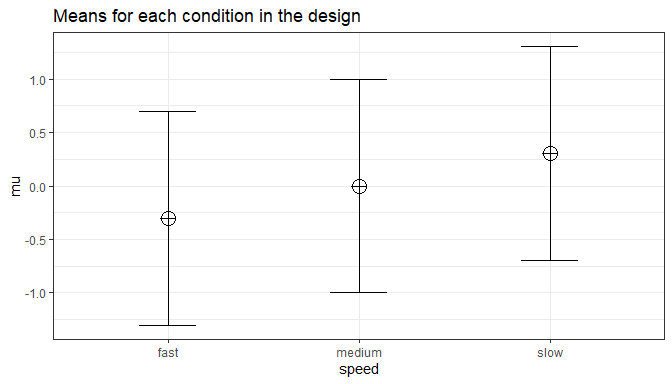# power_result_vig_3 <- ANOVA_power(design_result, nsims = nsims)
# saveRDS(power_result_vig_3, file = "sim_data/power_result_vig_3.rds")
power_result_vig_3 <- readRDS(file = "sim_data/power_result_vig_3.rds")

The results of the simulation are indeed very close to 96.9%. We can see this is in line with the power estimate from Gpower: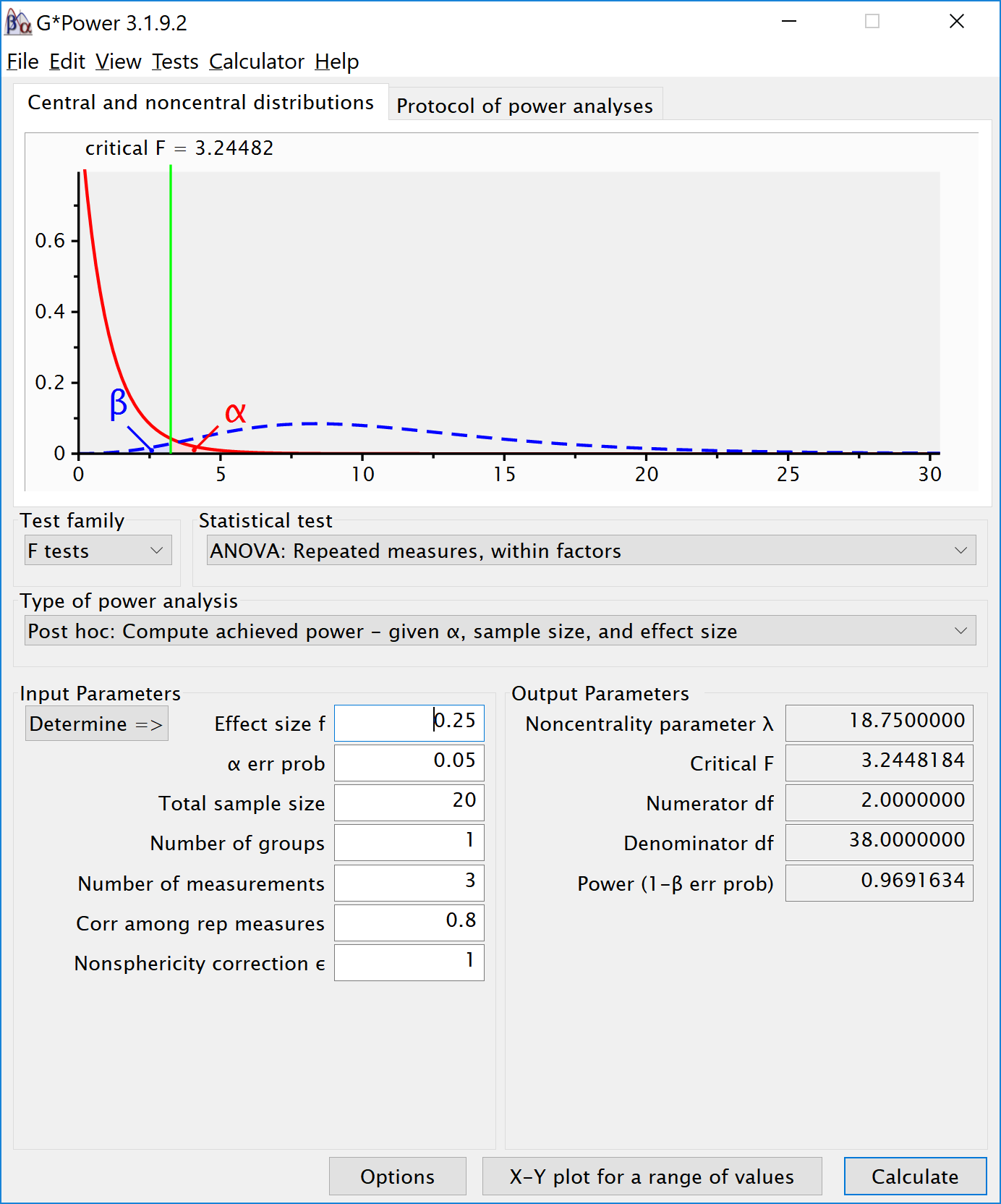We can even check the calculation of Cohen’s f SPSS style in GPower. We take the GPower settings as illustrated above. We click the ‘Options’ button, and check the radio button next to ‘As in SPSS’. Click ok, and you will notice that the ‘Corr among rep measures’ field has disappeared. The correlation does not need to be entered separately, but is incorporated in Cohen’s f. The value of Cohen’s f, which was 0.25, has changed into 0.7024394. This is the SPSS equivalent. The value is much larger. This value, and it’s corresponding partial eta-squared, incorporate the correlation between observations.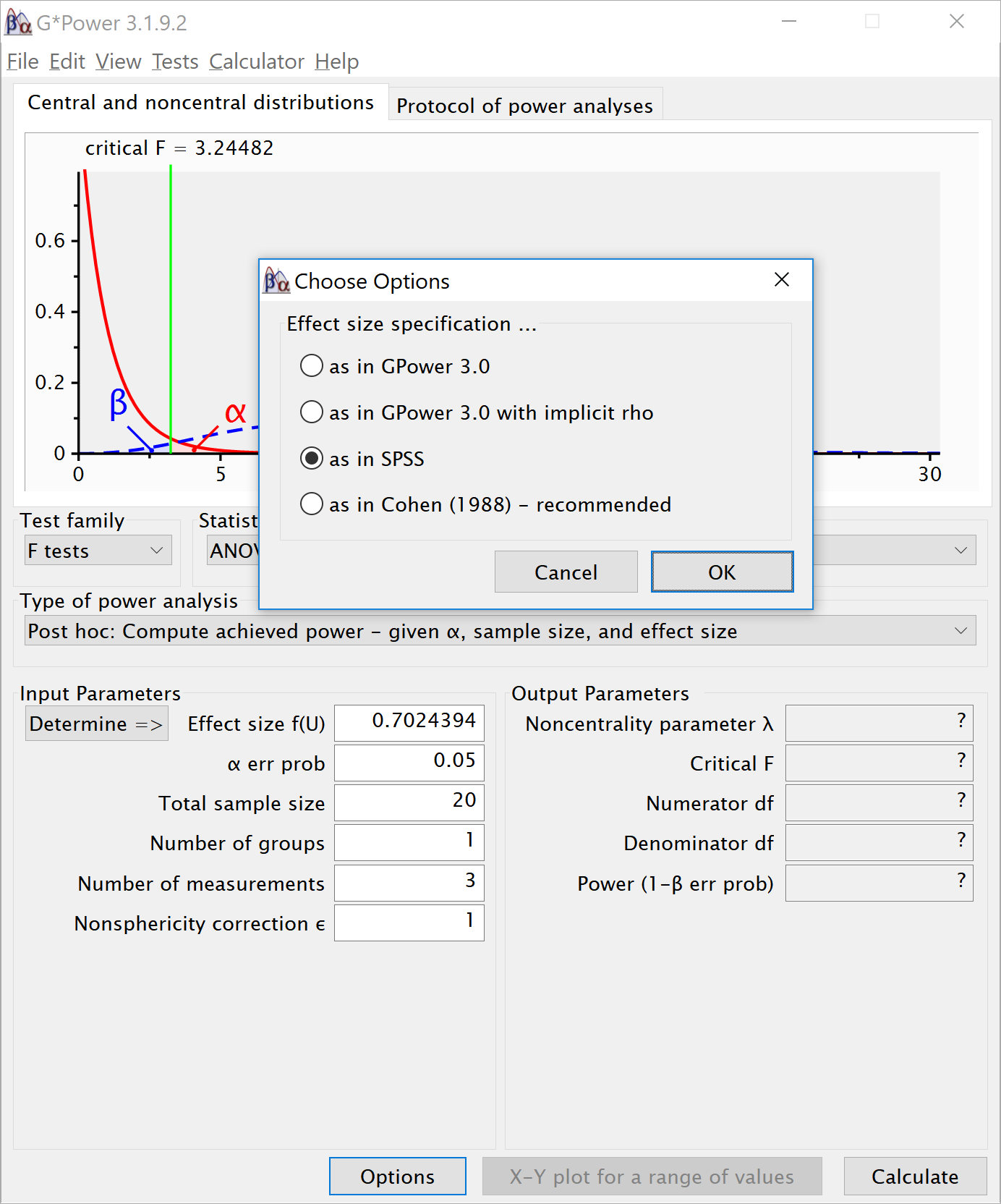## 2x2 ANOVA, within-between design

We can simulate a two-way ANOVA with a specific alpha, sample size and effect size, to achieve a specified statistical power. We will try to reproduce the power analysis in G*power for an F-test, ANOVA: Repeated measures, within-between interaction.